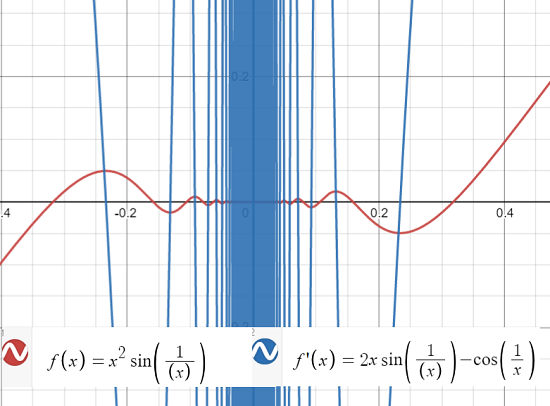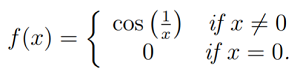# Darboux’s Theorem / Property

Darboux’s Theorem (also called Darboux Continuity or Darboux Property) tells us that any derivative has the Intermediate Value Theorem property, even discontinuous ones. The IVT says that if a continuous function takes on a negative value and then switches to a positive value, it must take on a value of “0” somewhere in between.

Up until French mathematician Jean Gaston Darboux developed the proof of his theorem in 1975, it was widely believed that the IVT implied continuity. Darboux showed this wasn’t the case, demonstrating several differentiable functions with discontinuous derivatives .

Darboux made several contributions to the theory of singularities in differential equations. The similarly-named “Darboux theorem” concerns neighborhoods of points in manifolds .

## Formal Definition of Darboux’s Theorem

Darboux’s Theorem is formally stated as :
Suppose a function is differentiable on a closed interval [a, b] so that:

• f′(a) = y1

and

• f′(b) = y2

If d lies between y1 and y2, then there is a point c in the interval [a, b] with f′(c) = d.

## Darboux’s Theorem ExampleThe above graph shows the function f(x) = x2 sin(1/x) and its discontinuous (at zero) derivative f′(x) = 2x sin(1/x) – cos(1/x). The problem with the derivative is that the second part, cos(1/x), isn’t defined at zero. But we can assign a value by tweaking the function’s definition:This perfectly valid definition means that the derivative now has the IVT property for certain intervals . For example [0.5, 2]. But it isn’t continuous, and behaves pathologically as it nears zero.

Many different proofs can be found in the literature. Several proofs of the theorem can be found in Dr. Mukta Bhandar’s paper Another Proof of Darboux’s Theorem available here.

## Darboux Property

The Darboux property is another name for Darboux’s theorem. However, some authors may use a slightly different definitions, which means that the two terms may in some cases also differ. For example, Beni Bogoşel states  that a function has the Darboux property if, for any interval I ⊂ ℝ, f(I) is also an interval; Bogoşel clarified in a comment that he was using a seldom-used form of the intermediate value property where the entire image is an interval. Continuity implies the Darboux property and the Darboux property implies the intermediate value property.

## References

 Olsen, L. A New Proof of Darboux’s Theorem. The American Mathematical Monthly Vol. 111, No. 8 (Oct., 2004), pp. 713-715. Retrieved April 14, 2021 from: https://www.jstor.org/stable/4145046?seq=1
 Lesfari, A. Moser’s lemma and the Darboux Theorem. Universal Journal of Applied Mathematics 2(1): 36-39, 2014. Retrieved April 14, 2021 from: https://citeseerx.ist.psu.edu/viewdoc/download?doi=10.1.1.1048.8405&rep=rep1&type=pdf
 Larson, R. & Edwards, B. (2016). Calculus, 10th Edition. Cengage Learning.
 Math 10850, Honors Calculus 1. (2018). Retrieved April 14, 2021 from: https://www3.nd.edu/~dgalvin1/10850/10850_F18/10850-tutorial_12.pdf
 Bhandar, M. Another Proof of Darboux’s Theorem. Retrieved April 14, 2021 from: https://arxiv.org/pdf/1601.02719.pdf
 Bogoşel, B. Problem list. Retrieved March 3, 2023 from: https://mathproblems123.wordpress.com/more-than-problems/problems/

CITE THIS AS:
Stephanie Glen. "Darboux’s Theorem / Property" From StatisticsHowTo.com: Elementary Statistics for the rest of us! https://www.statisticshowto.com/darbouxs-theorem-property/Need help with a homework or test question? With Chegg Study, you can get step-by-step solutions to your questions from an expert in the field. Your first 30 minutes with a Chegg tutor is free!